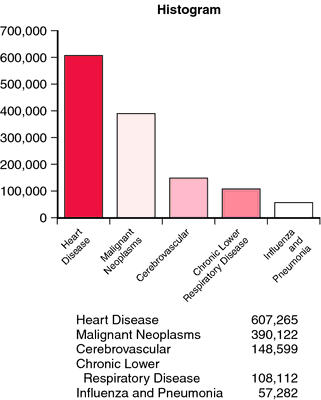histogram

(redirected from Frequency histogram)
Also found in: Dictionary, Thesaurus, Encyclopedia.

histogram

[his´to-gram]
a graph in which values found in a quantitative study are represented by lines or symbols placed horizontally or vertically, to indicate frequency distribution.Leading causes of death in the United States, 1999.

his·to·gram

(his'tō-gram),
1. A graphic columnar or bar representation to compare the magnitudes of frequencies or numbers of items.
2. Graphic representation of the frequency distribution of a variable, in which rectangles are drawn with their bases on a uniform linear scale representing intervals, and their heights are proportional to the values within each of the intervals.
[histo- + G. gramma, a writing]

his·to·gram

(his'tō-gram)
A bar chart representing a frequency distribution; heights of the bars indicate degree of frequency.
[histo- + G. gramma, a writing]

histogram

a diagram showing a frequency distribution by means of rectangular areas. See FREQUENCY DISTRIBUTION and Fig. 166 .

his·to·gram

(his'tō-gram)
Graphic columnar or bar representation to compare magnitudes of frequencies or numbers of items.
[histo- + G. gramma, a writing]
References in periodicals archive ?
Frequency histogram method in statistics was used to reveal the element compositions of calcium iron phase and calcium iron manganese phase in BOF slags.
Similarly, [G.sub.i](t)'s discrete density function can be expressed by frequency histogram. After getting frequency chart, we can use knowledge related to parameters estimation to estimate parameters of probability model.
The frequency histograms obtained by representative neurons can be fitted by a trimodal Gaussian distribution with data binned into 10 pA bins (Figures 3(b) and 3(c), right).
Frequency histogram of the 60 samples shows a positive skewness for element titanium (Fig.
Therefore vertical axis of Figure 1 that represents quality could be changed to represent frequency axis to plot frequency histograms of properties that possess the respective quality at the respective rental levels.
Tendencies for the decrease in the carriageway width are also indicated by the absolute frequency histogram. If the carriageway is too narrow, it is more complicated to pass through the roundabout, the heavyweight oversize vehicles are forced to ride up on the block pavement, and sometimes even on the road kerbs or pedestrian paths.
The resulting cumulative frequency histograms can be considered continuous variables, since the location and habitat values could range throughout the magnitude of the respective variables.
The colored digital image that has been produced by scanning the pavement surface was later converted to a grayscale image to generate a grayscale image frequency histogram. The pavement texture, then, has been classified based on the standard deviation of the frequency histogram.
In the framework of BOW method, each action video is represented as a k long vector, H = [[h.sub.l], ..., [h.sub.k]], namely, words frequency histogram, where [h.sub.j] is the number of times the nearest neighbor of a descriptor in the video is found to be [z.sub.j].
VIS Design 1: Frequency histograms. Based on the pre-computed results, the frequency histograms present distance, duration and start hour attributes of cycling behaviors.
The fracture orientations in each window are plotted on Schmidt nets, as shown in Figure 12; the frequency histograms (distributions) of the trace lengths and the apertures in each window are illustrated in Figures 13 and 14, respectively.
They were first calculated pixel by pixel for vegetated areas affected by the 2009/2010 drought quantified by DSI during the drought; then frequency histograms of the standardized anomalies of NDVI, EVI, NDWI, and LST were generated; finally, nonlinear curves of the frequency histograms of the standardized anomalies for NDVI, EVI, NDWI, and LST were fitted by a Gaussian function to show the skewed frequency distributions.

Site: Follow: Share:
Open / Close